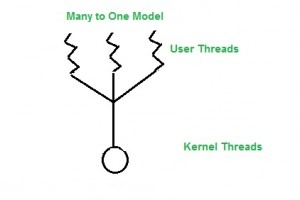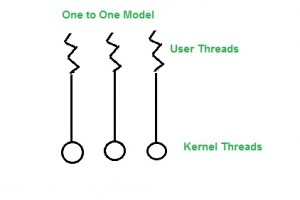# 9.2.1: Thread Types - Models

$$\newcommand{\vecs}{\overset { \rightharpoonup} {\mathbf{#1}} }$$ $$\newcommand{\vecd}{\overset{-\!-\!\rightharpoonup}{\vphantom{a}\smash {#1}}}$$$$\newcommand{\id}{\mathrm{id}}$$ $$\newcommand{\Span}{\mathrm{span}}$$ $$\newcommand{\kernel}{\mathrm{null}\,}$$ $$\newcommand{\range}{\mathrm{range}\,}$$ $$\newcommand{\RealPart}{\mathrm{Re}}$$ $$\newcommand{\ImaginaryPart}{\mathrm{Im}}$$ $$\newcommand{\Argument}{\mathrm{Arg}}$$ $$\newcommand{\norm}{\| #1 \|}$$ $$\newcommand{\inner}{\langle #1, #2 \rangle}$$ $$\newcommand{\Span}{\mathrm{span}}$$ $$\newcommand{\id}{\mathrm{id}}$$ $$\newcommand{\Span}{\mathrm{span}}$$ $$\newcommand{\kernel}{\mathrm{null}\,}$$ $$\newcommand{\range}{\mathrm{range}\,}$$ $$\newcommand{\RealPart}{\mathrm{Re}}$$ $$\newcommand{\ImaginaryPart}{\mathrm{Im}}$$ $$\newcommand{\Argument}{\mathrm{Arg}}$$ $$\newcommand{\norm}{\| #1 \|}$$ $$\newcommand{\inner}{\langle #1, #2 \rangle}$$ $$\newcommand{\Span}{\mathrm{span}}$$

## Multi Threading Models in Process Management

Many operating systems support kernel thread and user thread in a combined way. Example of such system is Solaris. Multi threading model are of three types.

Many to many model.
Many to one model.
one to one model.

### Many to Many Model

In this model, we have multiple user threads connected to the same or lesser number of kernel level threads. The number of kernel level threads are specific to the type of hardware. The advantage of this model is if a user thread is blocked for any reason, we can schedule others user threads to other kernel threads and the process itself continues to execute. Therefore, the entire process doesn’t block if a single thread is blocked.Figure $$\PageIndex{1}$$: Many to Many Thread Model. ("Many to Many" by Unknown, Geeks for Geeks is licensed under CC BY-SA 4.0)

Many to One Model

In this model, we have multiple user threads mapped to a single kernel thread. In this model if a user thread gets blocked by a system call, the process itself is blocked. Since we have only one kernel thread then only one user thread can access kernel at a time, so multiple user threads are not able access system calls at the same time.Figure $$\PageIndex{1}$$: Many to One Thread Model. ("Many to One" by Unknown, Geeks for Geeks is licensed under CC BY-SA 4.0)

One to One Model
In this model, there is a one to one relationship between kernel and user threads. Multiple thread can run on their own processor in a multiprocessor system. The problem with this model is that creating a user thread requires the creation of a kernel thread.Figure $$\PageIndex{1}$$: One to One Thread Model. ("One to One" by Unknown, Geeks for Geeks is licensed under CC BY-SA 4.0)